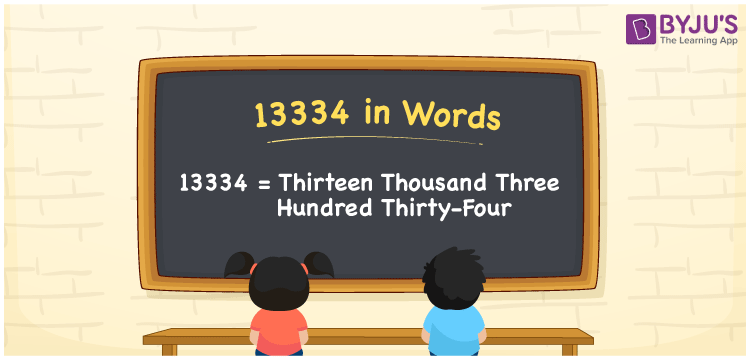# 13334 in words

13334 in words is written as Thirteen Thousand Three Hundred and Thirty Four. In 13334, 1 has a place value of ten thousand, the first 3 is in the place value of thousand, second 3 is in the place value of hundred, third 3 is in the place value of ten and 4 is in the place value of one. The article on Place Value gives more information. The number 13334 is used in expressions that relate to money, distance, social media views, and many more. For example, “There are Thirteen Thousand Three Hundred and Thirty Four people in this gated community.”

 13334 in words Thirteen Thousand Three Hundred and Thirty Four Thirteen Thousand Three Hundred and Thirty Four in Numbers 13334

## 13334 in English Words## How to Write 13334 in Words?

We can convert 13334 to words using a place value chart. The number 13334 has 5 digits, so let’s make a chart that shows the place value up to 5 digits.

 Ten thousand Thousands Hundreds Tens Ones 1 3 3 3 4

Thus, we can write the expanded form as:

1 × Ten thousand + 3 × Thousand + 3 × Hundred + 3 × Ten + 4 × One

= 1 × 10000 + 3 × 1000 + 3 × 100 + 3 × 10 + 4 × 1

= 13334.

= Thirteen Thousand Three Hundred and Thirty Four.

13334 is the natural number that is succeeded by 13333 and preceded by 13335.

13334 in words – Thirteen Thousand Three Hundred and Thirty Four.

Is 13334 an odd number? – No.

Is 13334 an even number? – Yes.

Is 13334 a perfect square number? – No.

Is 13334 a perfect cube number? – No.

Is 13334 a prime number? – No.

Is 13334 a composite number? – Yes.

## Solved Example

1. Write the number 13334 in expanded form

Solution: 1 x 10000 + 3 x 1000 + 3 x 100 + 3 x 10 + 4 x 1

We can write 13334 = 10000 + 3000 + 300 + 30 + 4

= 1 x 10000 + 3 x 1000 + 3 x 100 + 3 x 10 + 4 x 1.

## Frequently Asked Questions on 13334 in words

Q1

### How to write the number 13334 in words?

13334 in words is written as Thirteen Thousand Three Hundred and Thirty Four.
Q2

### Is 13334 a prime number?

No. 13334 is not a prime number.
Q3

### Is 13334 divisible by 10?

No. 13334 is not divisible by 10.